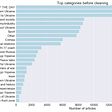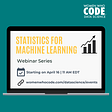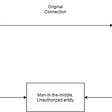# Confusion Matrix Role in Cyber Crime Detection

In this article, we all talk about cybercrime and its various types and finally, we will see how the confusion matrix helps to detect spam emails, which is a type of cybercrime.

# What is Cyber Crime?

Cybercrime is vastly growing in the world of tech today. Criminals of the World Wide Web exploit internet users’ personal information for their own gain. They dive deep into the dark web to buy and sell illegal products and services. They even gain access to classified government information.

Cybercrime is defined as a crime where a computer is the object of the crime or is used as a tool to commit an offense. A cybercriminal may use a device to access a user’s personal information, confidential business information, government information, or disable a device. It is also a cybercrime to sell or elicit the above information online.

# Some types of cybercrimes

• Email and internet fraud.
• Identity fraud.
• Theft of financial or card payment data.
• Theft and sale of corporate data.
• Cyberextortion (demanding money to prevent a threatened attack).
• Ransomware attacks (a type of cyber extortion).

Now we will see various steps involved in the classification of a email into two categories : either useful or spam. This classification accuracy will be measured with help of confusion matrix.

# Email Classification (binomial) :

So our problem is to classify the incoming emails into two categories of useful and spam. For this we are using the Spambase Data Set. In this dataset emails has 57 different independent features and using these features we have to classify the emails in two outcome categories : ‘spam’ & ‘normal’.

So first we will do all the pre processing of the dataset, and then we will build a classification model with preprocessed data.The last step in building a classification model is model scoring, which is based on comparing the actual and predicted target column values in the test set. The whole scoring process of a model consists of a match count: how many data rows have been correctly classified and how many data rows have been incorrectly classified by the model. These counts are summarized in the “confusion matrix”.

Here in email classification problem we have to find answers to the following questions:

• How many of the actual spam emails were predicted as spam?
• How many as normal?
• Were some normal emails predicted as spam?
• How many normal emails were predicted correctly?

These question will be answered with help of numbers shown in confusion matrix.And the class statistics are calculated on top of the confusion matrix.But before seeing the output accuracy through confusion matrix, let us first understand about the confusion matrix.

# Confusion Matrix :

The confusion matrix was initially introduced to evaluate results from binomial classification. Thus, the first thing to do is to take one of the two classes as the class of interest, i.e. the positive class. In the target column, we need to choose (arbitrarily) one value as the positive class. The other value is then automatically considered the negative class. This assignment is arbitrary, just keep in mind that some class statistics will show different values depending on the selected positive class.

So in our problem we chose the spam emails as the positive class and the normal emails as the negative class.

The confusion matrix gives count of four different numbers belonging to each class :

True Poitive (TP): The data rows (emails) belonging to the positive class (spam) and correctly classified as such.The number of true positives is placed in the top left cell of the confusion matrix.

False Negative (FN): The data rows (emails) belonging to the positive class (spam) and incorrectly classified as negative (normal emails).The number of false negatives is placed in the top right cell of the confusion matrix. It is also known as Type 2 error.

False Poitive (FP): The data rows (emails) belonging to the negative class (normal) and incorrectly classified as positive (spam emails).The number of false positives is placed in the lower left cell of the confusion matrix. It is also known as Type 1 error.

True Negative (TN): The data rows (emails) belonging to the negative class (normal) and correctly classified as such.The number of true negatives is placed in the lower right cell of the confusion matrix.

Hence, the correct predictions are on the diagonal with a gray background, the incorrect predictions are on the diagonal with a orange background:

## Mesaures to calculate model performance :

The four different counts in the confusion matrix, we can calculate a few class statistics measures to quantify the model performance.The class statistics summarizes the model performance for the positive and negative classes separately. To learn about these class statistics measure you can click here.

# Multivariate Email Classification model :

We can also use confusion matrix for multinomial classification model. suppose we have to classify emails into three categories such as ‘normal, ‘advertisment’ and ‘spam. So here also like in binomila classification, the target class values are assigned to the positive and the negative class. Here we define spam as the positive class and the normal and ad emails as the negative class.

Now, the confusion matrix will look something like this:

But unlike binomial classification, here we have to redefine the TP, FN, FP & TN in confusion matrix as :

True Poitive (TP): The cell identified by the row and column for the positive class contains the True Positives, i.e. where the actual and predicted class is spam

False Negative (FN): The Cells identified by the row for the positive class and columns for the negative class contain the False Negatives, where the actual class is spam, and the predicted class is normal or ad.

False Poitive (FP): The Cells identified by rows for the negative class and the column for the positive class contain the False Positives, where the actual class is normal or ad, and the predicted class is spam.

True Negative (TN): The Cells outside the row and column for the positive class contain the True Negatives, where the actual class is ad or normal, and the predicted class is ad or normal. An incorrect prediction inside the negative class is still considered as a true negative.

After this these four statistics will be used to calculate the model performance accuracy with the help of statistics measure discussed above.

# Conclusion :

• In this article, we have seen what is cybercrime and its various types.
• We have taken one cybercrime problem of spam emails to build a classification model for incoming emails to detect spam mails using confusion matrix.
• The confusion matrix shows the performance of a classification model: how many positive and negative events are predicted correctly or incorrectly. These counts are the basis for the calculation of more general class statistics metrics. Here, we reported those most commonly used: sensitivity and specificity, recall and precision, and the F-measure.
• Confusion matrix and class statistics have been defined for binomial classification problems. However, we have shown how they can be easily extended to address multinomial classification problems.

Thank you very much for reading the article!!!

--

--

--

## More from Abhishek Kumar

Love podcasts or audiobooks? Learn on the go with our new app.

## Bringing an Economist’s Perspective to Data Science with Susan Athey## Clearing “Mistry” Once for all: Data Engineer v/s Data Analyst v/s Data Scientist?## Monitoring the image of Ukraine in the media## WWCode Data Science Monthly Blog : April 2022## Demystify Feature Store## Brief Introduction to Clustering and different methods of Clustering## Data to Go: The Value of Data Portability as a Means to Data Liquidity## Geocoding and Reverse geocoding using Python## A Brief Introduction to Facial Recognition (Part 2)## Lidar sensing to improve safety## Watson Machine Learning & Studio Installation## A Brief Study on the Applications of Deep Learning in the Field of Information Security.